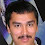## Wednesday, December 09, 2020

### Shuffling Around

In the fall of 1983 as a junior at Cornell I took CS 481, Introduction to the Theory of Computing, from Juris Hartmanis. Needless to say this was the course that changed my life and led me down a path that would have me teach a variation of this course myself more than twenty times.

For some reason one of the final exam questions is still stuck in my head.

Let the permutation of a language L be the set of strings x such that there is a string y in L which is a permutation of the letters in x. For example perm({01},{001})={01,10,001,010,100}.

Are regular languages closed under permutations?

There's a short and easy answer that's not so easy to find. Just in that P v NP spirit a Hartmanis test question should have. Give it a try before you read more.

If you ask Chegg you end up with

And for \$15 you can get the answer. Back in 1983 we called that cheating.

The hint only works if there are regular languages whose permutations are not context free. Which is true in general but oddly enough the permutation of every context-free language over a two-letter alphabet is context free. The proof uses Parikh's theorem which implies that the permutation of any context-free language is the permutation of a regular language (over any alphabet size).

Some other fun permutation questions:

• Show that NP is closed under permutations.
• Show that P is closed under permutations if and only if E = NE.
• What about NL and L?
Go ahead and chegg on those.

1.L=(10)* is regular, the permutation requires counting. Right?

1.I don't follow: The permutation of (10)* is (10)*, hence regular.

2.He's right. perm((10)*) is the language of strings with an equal number of 0s and 1s. The strings 0^n are distinguished by the strings 1^n. Myhill-Nerode: game over.

3.Oops.

I wonder how I make these mistakes. (01)* was my first thought but then somehow I began equating (01)* with * (in programming notation; aka (0+1)* in math notation), and I spent quite some time not realizing these are different. In hindsight, it doesn't make any sense.

Oh, well. I don't understand myself.

2."Why can't we have an open-book exam?"

"Because then I'd have to write a question so complex no book would have the answer."

3.Another recipe to prove P != NP